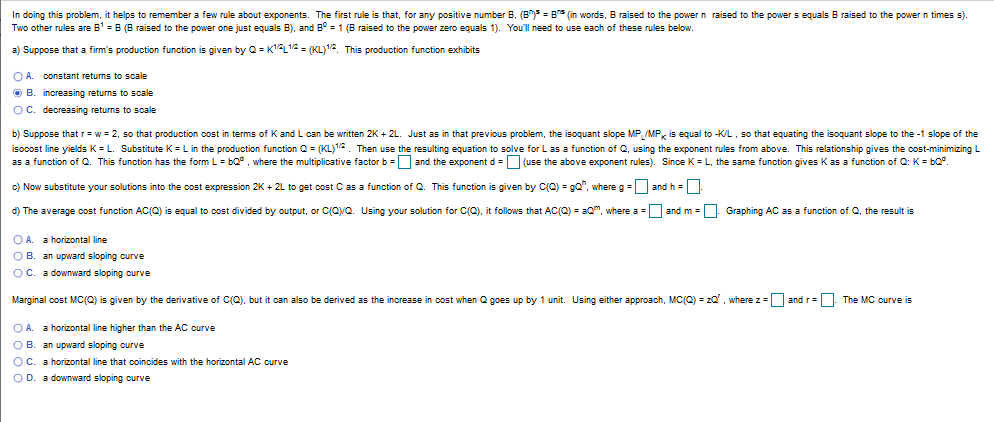# In doing this problem, it helps to remember a few rule about exponents. The first rule is that, for any positive number B, (B B (in words, B raised to the power n raised to the power s equals B raised to the power n times s).Two other rules are B B (B raised to the power one just equals B), and B1 (B raised to the power zero equals 1). You'll need to use each of these rules belowK1L1/2= ( KL) 12a) Suppose that a firm's production function is given by QThis production function exhibitsO A. constant returns to scaleB. increasing returns to scaleO C. decreasing returns to scaleb) Suppose that r w 2, so that production cost in terms of K and L can be written 2K+ 2L. Just as in that previous problem, the isoquant slope MP, MP is equalisocost line yields K L. Substitute K L in the production function Q (KL)1. Then use the resulting equation to solve for L as a function of Q, using the exponent rules from above. This relations hip gives the cost-minimizing Las a function of Q. This function has the form L bQwhere the multiplicative factor b |and the exponent d (use the above exponent rules). Since K L, the same function gives K as a function of Q: K bQ-KL, so that equating the isoquant slope to the -1 slope of thec) Now substitute your solutions into the cost expression 2K+ 2L to get cost C as a function of Q. This function is given by C(Q) gQ", where gand h =d) The average cost function AC(0) is equal to cost divided by output, or C(QyQ. Using your solution for C(Q), it follows that AC(Q) am where a and mGraphing AC as a function of Q, the result isO A.a horizontal lineO B. an upward sloping ourveO C. a downward sloping curveMarginal cost MC(Q) is given by the derivative of C(Q), but it can also be derived as the increase in cost when Q goes up by 1 unit. Using either approach, MC(Q) zand r=The MC curve iswhere zO A. a horizontal line higher than the AC curveO B. an upward sloping ourveO C. a horizontal line that coincides with the horizontal AC curveO D. a downward sloping ourve

Question
6 viewshelp_outlineImage TranscriptioncloseIn doing this problem, it helps to remember a few rule about exponents. The first rule is that, for any positive number B, (B B (in words, B raised to the power n raised to the power s equals B raised to the power n times s). Two other rules are B B (B raised to the power one just equals B), and B1 (B raised to the power zero equals 1). You'll need to use each of these rules below K1L1/2= ( KL) 12 a) Suppose that a firm's production function is given by Q This production function exhibits O A. constant returns to scale B. increasing returns to scale O C. decreasing returns to scale b) Suppose that r w 2, so that production cost in terms of K and L can be written 2K+ 2L. Just as in that previous problem, the isoquant slope MP, MP is equal isocost line yields K L. Substitute K L in the production function Q (KL)1. Then use the resulting equation to solve for L as a function of Q, using the exponent rules from above. This relations hip gives the cost-minimizing L as a function of Q. This function has the form L bQwhere the multiplicative factor b |and the exponent d (use the above exponent rules). Since K L, the same function gives K as a function of Q: K bQ -KL, so that equating the isoquant slope to the -1 slope of the c) Now substitute your solutions into the cost expression 2K+ 2L to get cost C as a function of Q. This function is given by C(Q) gQ", where g and h = d) The average cost function AC(0) is equal to cost divided by output, or C(QyQ. Using your solution for C(Q), it follows that AC(Q) am where a and m Graphing AC as a function of Q, the result is O A. a horizontal line O B. an upward sloping ourve O C. a downward sloping curve Marginal cost MC(Q) is given by the derivative of C(Q), but it can also be derived as the increase in cost when Q goes up by 1 unit. Using either approach, MC(Q) z and r=The MC curve is where z O A. a horizontal line higher than the AC curve O B. an upward sloping ourve O C. a horizontal line that coincides with the horizontal AC curve O D. a downward sloping ourve fullscreen
check_circle

Step 1

(a)

If the capital and the labor increased by k times. The production function can be derived as follows.

Step 2

Here, the output is multiplied with K and the labor and capital is also multiplied by K. Thus, we can say that the production function shows a constant return to scale.

Step 3

(b)

Suppose,

Wage and the rental rate = 2.

Production cost = 2K+2L.

Isoquant slope = -K/L.

Isocost slop = -...

### Want to see the full answer?

See Solution

#### Want to see this answer and more?

Solutions are written by subject experts who are available 24/7. Questions are typically answered within 1 hour.*

See Solution
*Response times may vary by subject and question.
Tagged in